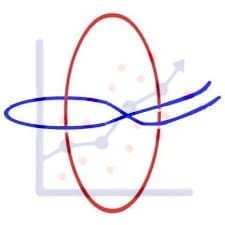Get inspired by the success stories of our students in IIT JAM MS, ISI  MStat, CMI MSc Data Science.  Learn More

# ISI MStat PSB 2005 Problem 2 | Calculating probability using Binomial DistributionThis is a very beautiful sample problem from ISI MStat PSB 2005 Problem 2 based on finding probability using the binomial distribution. Let's give it a try !!

## Problem- ISI MStat PSB 2005 Problem 2

Let $X$ and $Y$ be independent random variables with X having a binomial distribution with parameters 5 and $1 / 2$ and $Y$ having a binomial distribution with parameters 7 and $1 / 2 .$ Find the probability that $|X-Y|$ is even.

### Prerequisites

Binomial Distribution

Binomial Expansion

Parity Check

## Solution :

Given $X \sim$ Bin(5,1/2) and $Y \sim$ Bin(7,1/2) , and they are independent .

Now , we have to find , $P(|X-Y|=even )$

$|X-Y|$= even if both X and Y are even or both X and Y are odd .

Therefore $P(|X-Y|=even )=P(X=even,Y=even) + P(X=odd , Y=odd)$

P(X=even , Y= even ) =$( {5 \choose 0} {(\frac{1}{2})}^5 + {5 \choose 2} {(\frac{1}{2})}^5 + \cdots + {5 \choose 4} {(\frac{1}{2})}^5 )( {7 \choose 0} {(\frac{1}{2})}^7 + {7 \choose 2} {(\frac{1}{2})}^7 + \cdots + {7 \choose 6} {(\frac{1}{2})}^7)$

=$({(\frac{1}{2})}^5 \times \frac{2^5}{2})({(\frac{1}{2})}^7 \times \frac{2^7}{2})$

= $\frac{1}{4}$

Similarly , one can find P(X=odd , Y=odd ) which is coming out to be $\frac{1}{4}$ .

Hence , P(|X-Y|) = 14+1/4 = 1/2 .

## Food For Thought

Try to find P(X-Y=odd) under the same condition as given in the above problem .

## Subscribe to Cheenta at Youtube

This is a very beautiful sample problem from ISI MStat PSB 2005 Problem 2 based on finding probability using the binomial distribution. Let's give it a try !!

## Problem- ISI MStat PSB 2005 Problem 2

Let $X$ and $Y$ be independent random variables with X having a binomial distribution with parameters 5 and $1 / 2$ and $Y$ having a binomial distribution with parameters 7 and $1 / 2 .$ Find the probability that $|X-Y|$ is even.

### Prerequisites

Binomial Distribution

Binomial Expansion

Parity Check

## Solution :

Given $X \sim$ Bin(5,1/2) and $Y \sim$ Bin(7,1/2) , and they are independent .

Now , we have to find , $P(|X-Y|=even )$

$|X-Y|$= even if both X and Y are even or both X and Y are odd .

Therefore $P(|X-Y|=even )=P(X=even,Y=even) + P(X=odd , Y=odd)$

P(X=even , Y= even ) =$( {5 \choose 0} {(\frac{1}{2})}^5 + {5 \choose 2} {(\frac{1}{2})}^5 + \cdots + {5 \choose 4} {(\frac{1}{2})}^5 )( {7 \choose 0} {(\frac{1}{2})}^7 + {7 \choose 2} {(\frac{1}{2})}^7 + \cdots + {7 \choose 6} {(\frac{1}{2})}^7)$

=$({(\frac{1}{2})}^5 \times \frac{2^5}{2})({(\frac{1}{2})}^7 \times \frac{2^7}{2})$

= $\frac{1}{4}$

Similarly , one can find P(X=odd , Y=odd ) which is coming out to be $\frac{1}{4}$ .

Hence , P(|X-Y|) = 14+1/4 = 1/2 .

## Food For Thought

Try to find P(X-Y=odd) under the same condition as given in the above problem .

## Subscribe to Cheenta at Youtube

This site uses Akismet to reduce spam. Learn how your comment data is processed.

### Knowledge Partner